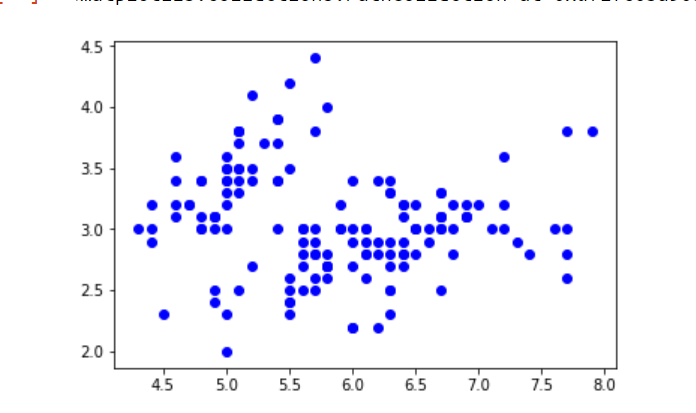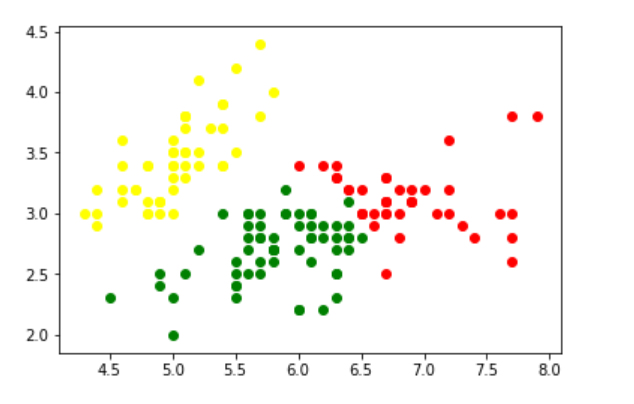GFG App
Open AppBrowser
Continue

Suppose there are a set of data points that need to be grouped into several parts or clusters based on their similarity. In Machine Learning, this is known as Clustering. There are several methods available for clustering:

### Normal or Gaussian Distribution

In real life, many datasets can be modeled by Gaussian Distribution (Univariate or Multivariate). So it is quite natural and intuitive to assume that the clusters come from different Gaussian Distributions. Or in other words, it tried to model the dataset as a mixture of several Gaussian Distributions. This is the core idea of this model.
In one dimension the probability density function of a Gaussian Distribution is given bywhereandare respectively the mean and variance of the distribution. For Multivariate ( let us say d-variate) Gaussian Distribution, the probability density function is given byHereis a d dimensional vector denoting the mean of the distribution andis the d X d covariance matrix.

### Gaussian Mixture Model

Suppose there are K clusters (For the sake of simplicity here it is assumed that the number of clusters is known and it is K). Soandare also estimated for each k. Had it been only one distribution, they would have been estimated by the maximum-likelihood method. But since there are K such clusters and the probability density is defined as a linear function of densities of all these K distributions, i.e.whereis the mixing coefficient for kth distribution. For estimating the parameters by the maximum log-likelihood method, compute p(X|).Now define a random variablesuch that=p(k|X).

From Bayes theorem,Now for the log-likelihood function to be maximum, its derivative ofwith respect to, andshould be zero. So equating the derivative ofwith respect toto zero and rearranging the terms,Similarly taking the derivative with respect toand pi respectively, one can obtain the following expressions.AndNote:denotes the total number of sample points in the kth cluster. Here it is assumed that there is a total N number of samples and each sample containing d features is denoted by.

So it can be clearly seen that the parameters cannot be estimated in closed form. This is where the Expectation-Maximization algorithm is beneficial.

### Expectation-Maximization (EM) Algorithm

The Expectation-Maximization (EM) algorithm is an iterative way to find maximum-likelihood estimates for model parameters when the data is incomplete or has some missing data points or has some hidden variables. EM chooses some random values for the missing data points and estimates a new set of data. These new values are then recursively used to estimate a better first date, by filling up missing points, until the values get fixed.

These are the two basic steps of the EM algorithm, namely the E Step, or Expectation Step or Estimation Step, and M Step, or Maximization Step.

#### Estimation step

Initializeandby some random values, or by K means clustering results or by hierarchical clustering results. Then for those given parameter values, estimate the value of the latent variables (i.e)

#### Maximization Step

Update the value of the parameters( i.e.and) calculated using the ML method.

### Implementation of the Gaussian Mixture Model

In this example, iris Dataset is taken. In Python, there is a Gaussian mixture class to implement GMM. Load the iris dataset from the datasets package. To keep things simple, take the only first two columns (i.e sepal length and sepal width respectively). Now plot the dataset.

## Python3

 import numpy as np import pandas as pd import matplotlib.pyplot as plt from pandas import DataFrame from sklearn import datasets from sklearn.mixture import GaussianMixture   # load the iris dataset iris = datasets.load_iris()   # select first two columns  X = iris.data[:, :2]   # turn it into a dataframe d = pd.DataFrame(X)   # plot the data plt.scatter(d, d) plt.show()

Output:Now fit the data as a mixture of 3 Gaussians. Then do the clustering, i.e assign a label to each observation. Also, find the number of iterations needed for the log-likelihood function to converge and the converged log-likelihood value.

## Python3

 gmm = GaussianMixture(n_components = 3)   # Fit the GMM model for the dataset  # which expresses the dataset as a  # mixture of 3 Gaussian Distribution gmm.fit(d)   # Assign a label to each sample labels = gmm.predict(d) d['labels']= labels d0 = d[d['labels']== 0] d1 = d[d['labels']== 1] d2 = d[d['labels']== 2]   # plot three clusters in same plot plt.scatter(d0, d0, c ='r') plt.scatter(d1, d1, c ='yellow') plt.scatter(d2, d2, c ='g') plt.show()

Output:Print the converged log-likelihood value and no. of iterations needed for the model to converge

## Python3

 # print the converged log-likelihood value print(gmm.lower_bound_)   # print the number of iterations needed # for the log-likelihood value to converge print(gmm.n_iter_)

Output:

-1.4985672470486966
8

Hence, it needed 7 iterations for the log-likelihood to converge. If more iterations are performed, no appreciable change in the log-likelihood value can be observed.

My Personal Notes arrow_drop_up